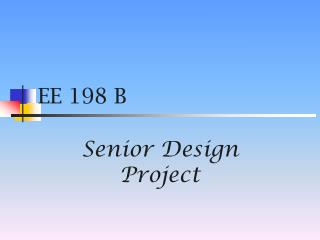# EE 198 B - PowerPoint PPT PresentationDownload PresentationEE 198 B

EE 198 B
Download Presentation## EE 198 B

- - - - - - - - - - - - - - - - - - - - - - - - - - - E N D - - - - - - - - - - - - - - - - - - - - - - - - - - -
##### Presentation Transcript

1. EE 198 B SeniorDesign Project

2. Spectrum Analyzer

3. Designed by • Archanna Srinivasan • Shweta Upadhyaya • Nelson Chen • Wing Chan • Xingli Zhu

4. Details • Advisor: Professor Freeman • Date of Presentation: May 14th 2004 • San Jose State University

5. Special Thanks to: • Professor Freeman • Professor Strasilla • Ms. Irma Alarcon • NI Representatives

6. Purpose of the Project • To implement a Spectrum Analyzer in LabVIEW Software that is cost efficient.

7. Definition of an Analyzer • Analyzes the spectral content (amplitude and frequency) of the input signal. • Analyzes the various harmonics present in the signal.

8. Application • Used to measure signal characteristics such as carrier level, sidebands, harmonics, and phase noise. • Used to measure the spectral purity of multiplex signals and the percentage of modulation of AM signals. • Can analyze distortion and spurious signals, and can determine the non-linearity in a system.

9. Specifications: • Input Frequency: 0-500 KHz • Dc Voltage Maximum: +/- 5 V amplitude • Number of Bits = 8 bits • Resolution: 0.04 V • FS: Sampling Frequency = 20 MHz • Number of Harmonics used = 3

10. BLOCK DIAGRAM OF THE SYSTEM Function Generator Power Supply Circuit Board NI PCI 5102 Digitizer COMPUTER Pentium 4 256 MB RAM Oscilloscope

11. Block Diagram of Software Input Low Pass Filter Amplitude & Level Measurement FFT (Spectral) Distortion Measurement Tone Measurement Write LabVIEW

12. Analysis of the Blocks

13. Block Diagram of the Input NI Scope Initialize NI Scope Auto Setup NI Scope Multi Read NI Scope Close Error Handler Index Array Un bundle by Name Build Waveform Convert to Dynamic Data Input to the Express VI’s

14. Input • A cluster of waveforms are accessed. • The waveforms are separated out into indices in an array. • A single waveform is extracted and expanded to three signals wide. • The single resulting waveform is converted to dynamic data for use by the Express VI’s.

15. Low pass Filter • Why Butterworth? • It has a smooth response at all frequencies and it is maximally flat. • Why order 3? • High order is chosen to give more accuracy.

16. Fast Fourier Transform (FFT) • Main calculation block for the output. • Displays the frequency response - Vrms (dB) versus frequency. • Also displays the phase in degrees or radians.

17. Harmonic Distortion Measurement Block • Integral multiples of the fundamental frequency (f): 2f, 3f, 4f...and so on are called harmonics. • The higher the number and amplitude of the harmonics, the more distorted is the system. • Helps to measure the non-linearity of a system.

18. Distortion Parameters • THD: is the sum of the powers of all harmonic frequencies above the fundamental frequency to the power of the fundamental frequency. • Specific Harmonic: returns the value of the harmonic that is specified, in this case it is three.

19. Tone Measurements • Finds the single tone with the highest amplitude. • Searches a specified frequency range to find the single tone with the highest amplitude. • All outputs (Amplitude, Frequency and Phase)have numeric displays.

20. Amplitude/Level Measurements • Performs voltage measurements and analysis on the input signal. • Returns the DC voltage, AC Positive Peak and Negative Peak, Peak to Peak voltage, RMS (root mean square) of the input signal. • Above parameters have numeric display at the front panel.

21. Write LabVIEW • Writes data to a LabVIEW data measurement file. • It receives input from Spectrum Measurement Block’s FFT. • The File Name and Comments Input are entered through Dialog Box by the user. • The outputs are File Name, Saving Data. • This file can be accessed using Microsoft Excel.

22. How is ours Better? • The input from the NI-Scope Digitizer was made compatible with the LabVIEW Express VI’s for the first time ever.

23. Cost Analysis • How is our project cost-effective?

24. Cost Comparison • HP/Agilent 860E Spectrum Analyzer (input frequency: 30hz – 2.9Ghz) sold at \$27,530 • Our cost • DAQ Card + Probes = \$1500 • Computer = \$900 • Software = Free…Student Version • Total = \$2400

25. Examples • Low Pass Filter

26. First Order Low Pass Filter

27. Common Emitter Amplifier

28. Common Emitter Gain vs. Freq (Scope & Analyzer)

29. Demo • High Pass Filter

30. Testing Spectrum Analyzer First order high pass filter using LM741 Op-Amp. Compare results taken from the Spectrum Analyzer with results from the Oscilloscope. Resulting graphs are plotted in Excel.

31. High Pass Filter Circuit

32. High pass filter Excel graph:

33. Illustration of Non-Idealities in an Op-amp

34. Results Found • The graphs from the Oscilloscope and the Spectrum Analyzer follows the same shape. • They follow the same characteristics of a first order high pass active filter using LM741 Op Amp. • Data from the scope are taken using the scope itself, while data from the Spectrum Analyzer are taken from the FFT graph. • As the frequency of a High Pass increases, the performance of the filter degrades as shown in the graph.

35. QUESTIONS???